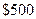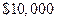Chapter 17, Problem 7SCQ### Principles of Economics 2e

2nd Edition
Steven A. Greenlaw; David Shapiro
ISBN: 9781947172364

#### Solutions

Chapter
Section### Principles of Economics 2e

2nd Edition
Steven A. Greenlaw; David Shapiro
ISBN: 9781947172364
Textbook Problem

# If you receivein simple interest on a loan that you made forfor five years, what was the interest rate you charged?

To determine

Calculation of interest rate.

Explanation

The interest rate charged is 1 percent.

Simple Interest = Prinicpal × Rate × TimeInterest amount = $500Time period = 5 yearPrincipal amount =$10000Interest Rate = ?I

### Still sussing out bartleby?

Check out a sample textbook solution.

See a sample solution

#### The Solution to Your Study Problems

Bartleby provides explanations to thousands of textbook problems written by our experts, many with advanced degrees!

Get Started